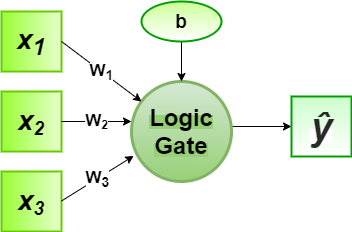# Perceptron Algorithm for Logic Gate with 3-bit Binary Input

• Last Updated : 18 Aug, 2020

In the field of Machine Learning, the Perceptron is a Supervised Learning Algorithm for binary classifiers. The Perceptron Model implements the following function:For a particular choice of the weight vectorand bias parameter, the model predicts outputfor the corresponding input vector.
The logical function truth table of AND, OR, NAND, NOR gates for 3-bit binary variables, i.e, the input vectorand the corresponding output0000011
0010110
0100110
0110110
1000110
1010110
1100110
1111100

Now for the corresponding weight vectorof the input vector, the associated Perceptron Function can be defined as:For the implementation, considered weight parameters areand the bias parameter isfor every logic gates-11-1-111-1-111-1-1-2-0.931

Python Implementation:

 # importing python libraryimport numpy as np  # sigmoid activation functiondef activationFunction(model, type ="sigmoid"):   return {       "sigmoid": 1 / (1 + np.exp(-model))   }[type]  # designing perceptron modeldef perceptronModel(weights, inputs, bias):   model = np.add(np.dot(inputs, weights), bias)   logic = activationFunction(model, type ="sigmoid")   return np.round(logic)  # computation modeldef compute(data, logicGate, weights, bias):   weights = np.array(weights)   output = np.array([ perceptronModel(weights,              datum, bias) for datum in data ])   return output  # Print Outputdef printOutput(dataset, name, data):   print("Logic Function: {}".format(name.upper()))   print("X1\tX2\tX3\tY")   toPrint = ["{1}\t{2}\t{3}\t{0}".format(output, *datas)                for datas, output in zip(dataset, data)]   for i in toPrint:       print(i)  # main functiondef main():   # 3 bit binary data   dataset = np.array([     [0, 0, 0],     [0, 0, 1],     [0, 1, 0],     [0, 1, 1],     [1, 0, 0],     [1, 0, 1],     [1, 1, 0],     [1, 1, 1]   ])     # Parameters of every Logic Gates   # weight parameters: w1, w2, w3   # bias parameter: b   logicGate = {       "and": compute(dataset, "and", [1, 1, 1], -2),       "or": compute(dataset, "or", [1, 1, 1], -0.9),       "nand": compute(dataset, "nand", [-1, -1, -1], 3),       "nor": compute(dataset, "nor", [-1, -1, -1], 1)   }   for gate in logicGate:       printOutput(dataset, gate, logicGate[gate])  if __name__ == '__main__':   main()
Output:
Logic Function: AND
X1    X2    X3    Y
0    0    0    0.0
0    0    1    0.0
0    1    0    0.0
0    1    1    0.0
1    0    0    0.0
1    0    1    0.0
1    1    0    0.0
1    1    1    1.0
Logic Function: OR
X1    X2    X3    Y
0    0    0    0.0
0    0    1    1.0
0    1    0    1.0
0    1    1    1.0
1    0    0    1.0
1    0    1    1.0
1    1    0    1.0
1    1    1    1.0
Logic Function: NAND
X1    X2    X3    Y
0    0    0    1.0
0    0    1    1.0
0    1    0    1.0
0    1    1    1.0
1    0    0    1.0
1    0    1    1.0
1    1    0    1.0
1    1    1    0.0
Logic Function: NOR
X1    X2    X3    Y
0    0    0    1.0
0    0    1    0.0
0    1    0    0.0
0    1    1    0.0
1    0    0    0.0
1    0    1    0.0
1    1    0    0.0
1    1    1    0.0


Here, the model predicted output () for each of the test inputs are exactly matched with the AND, OR, NAND, NOR logic gates conventional output ()s according to the truth table for 3-bit binary input.
Hence, it is verified that the perceptron algorithm for all these logic gates is correctly implemented.

My Personal Notes arrow_drop_up